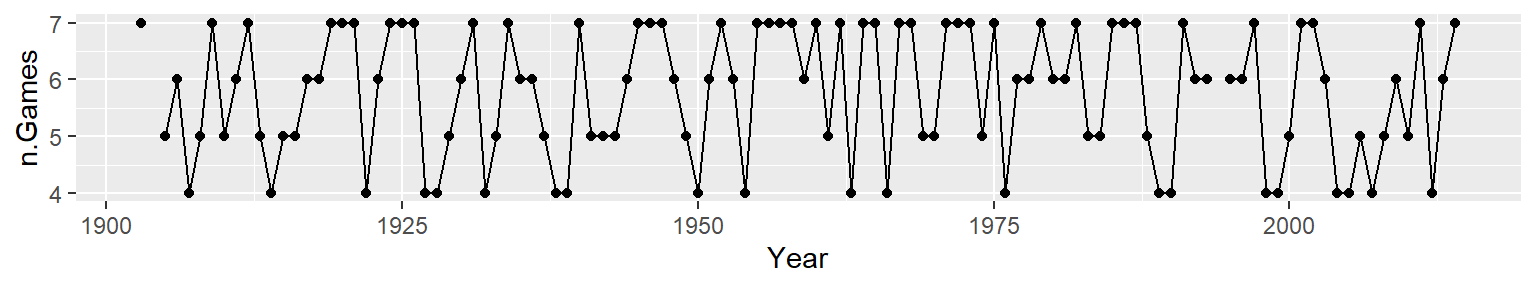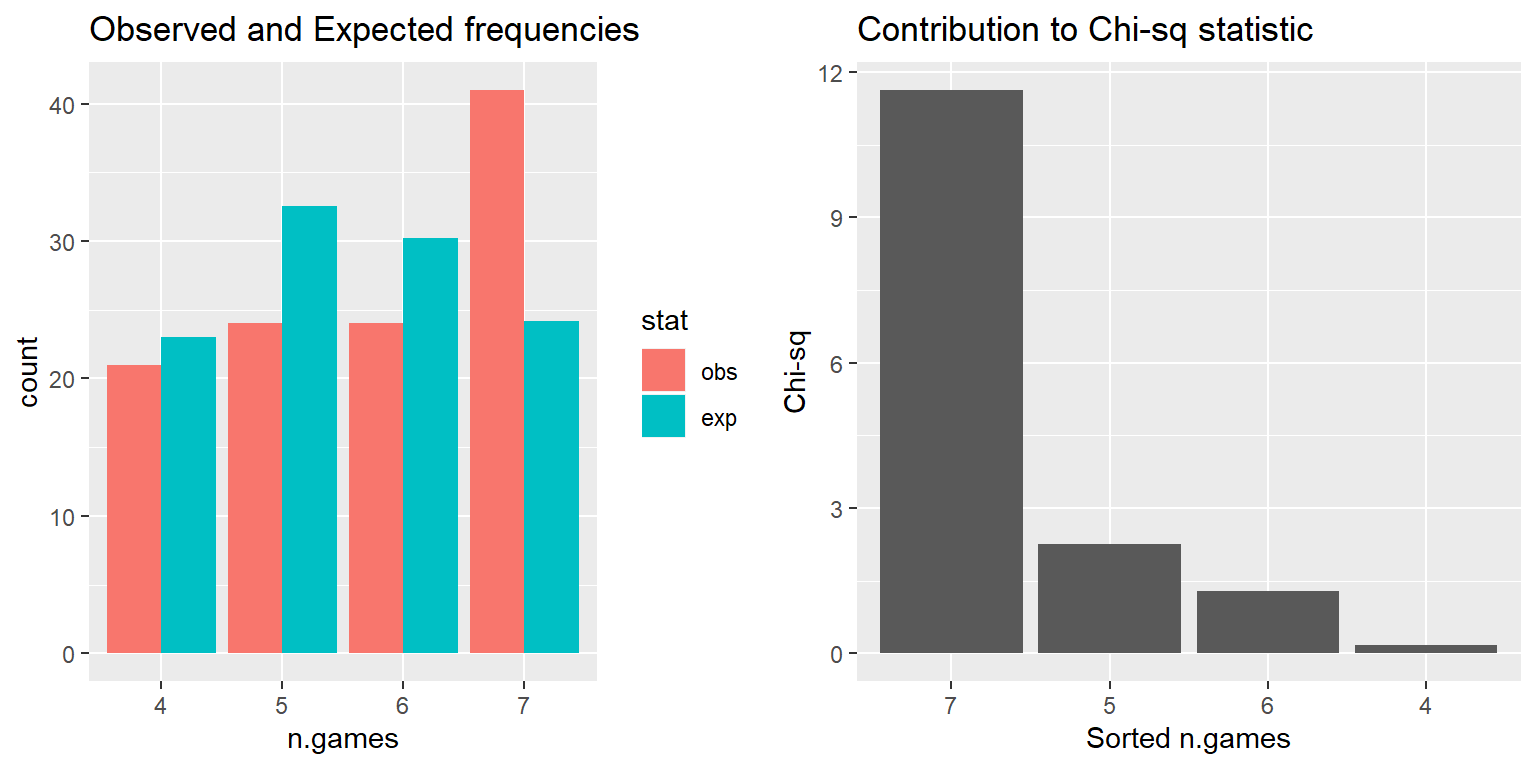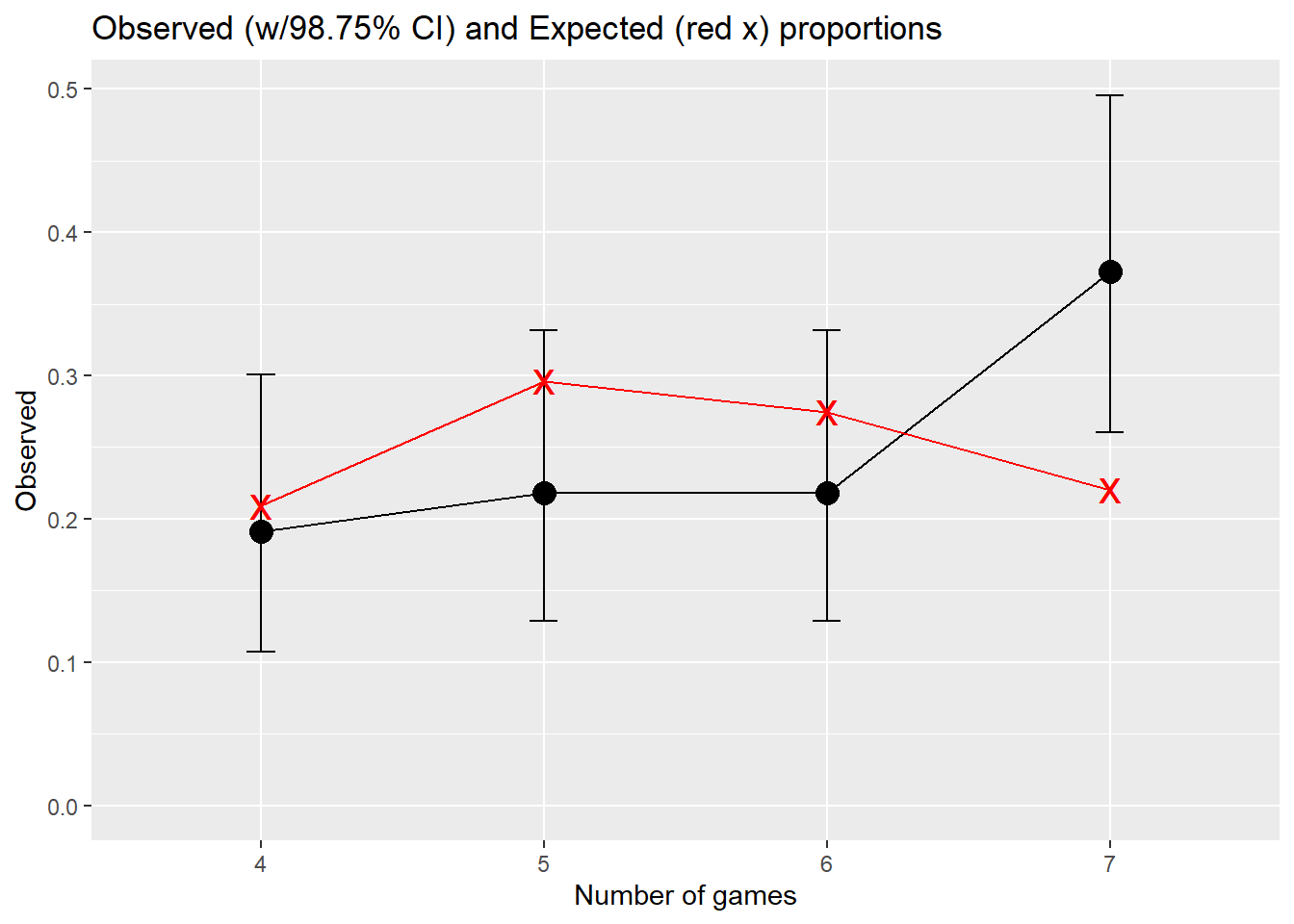Include your answers in this document in the sections below the rubric where I have point values indicated (1 p).

# Rubric

Answer the questions with the data example.

# World Series expected number of games

One form of sportsball is the game of Baseball. Its championship, played exclusively in North America, is called the “World Series”.

How well does the distribution of number of games played during the Baseball World Series follow the expected distribution? This is a multinomial goodness-of-fit test. There are four categories for the number of games (4, 5, 6, and 7) and for each World Series the number of games must fall into one of these categories.

## Calculate observed and expected frequencies

We read in a table summarizing the World Series history, and calculate the total number of games played.

# read data
mlb.ws <- read.csv(fn.dat, skip = 1, stringsAsFactors = FALSE)
head(mlb.ws)
##   Year      Winning.team       Manager League Games            Losing.team
## 1 1903  Boston Americans Jimmy Collins     AL   5-3      Pittsburg Pirates
## 2 1904   No World Series             -      -     -                      -
## 3 1905   New York Giants   John McGraw     NL   4-1 Philadelphia Athletics
## 4 1906 Chicago White Sox Fielder Jones     AL   4-2           Chicago Cubs
## 5 1907      Chicago Cubs  Frank Chance     NL   4-0         Detroit Tigers
## 6 1908      Chicago Cubs  Frank Chance     NL   4-1         Detroit Tigers
##       Manager.1 League.1 Ref.
## 1   Fred Clarke       NL  
## 2             -        -  
## 3   Connie Mack       AL 
## 4  Frank Chance       NL 
## 5 Hugh Jennings       AL 
## 6 Hugh Jennings       AL 
# split "Games" into win/lose games, convert to numeric, add, and vectorize
library(stringr)
# this is one line, but I've formatted it so you can see
#   what it's doing from the inside outward

# 6. assign this to a new column for number of games
mlb.ws$n.Games <- # 5. convert the vector to numeric as.numeric( # 4. collapse the lists into one vector cbind( # 3. add the elements in each list element lapply( # 2. convert each list element to numeric lapply( # 1. split Games at the hyphen # this creates list of lists, # each small list has the two values # that were split by the hyphen str_split(mlb.ws$Games, "-")
, as.numeric
)
, sum)
)
)

## in practice, you might write this as a single line like this,
##   but when you do it this way, it takes a while for someone else
##   to understand what you're doing, or why, or how.
# mlb.ws$n.Games <- as.numeric(cbind(lapply(lapply(str_split(mlb.ws$Games, "-"), as.numeric), sum)))

Note that the 1903, 1919, 1920, and 1921 World Series were in a best-of-nine format (carried by the first team to win five games). When more than 7 games were played, I assigned those to 7 games.

# replace games > 7 with 7 games
mlb.ws$n.Games[(mlb.ws$n.Games > 7)] <- 7

### Observed

Here’s the observed number of games over the history of the World Series.

library(ggplot2)
p <- ggplot(mlb.ws, aes(x = Year, y = n.Games, group = 1))
p <- p + geom_point()
p <- p + geom_line()
print(p)
## Warning: Removed 2 rows containing missing values (geom_point).# observed proportions
obs.games <- table(mlb.ws$n.Games) obs.games ## ## 4 5 6 7 ## 21 24 24 41 prop.table(obs.games) ## ## 4 5 6 7 ## 0.1909091 0.2181818 0.2181818 0.3727273 ### Expected Under an assumption of the probability of a particular team winning a single game, we can calculate the probability of 4, 5, 6, or 7 games, and therefore the expected number of World Series ending with each number of games. (1 p) Below, set your own probability of a particular team winning each game. On average, one team is a little better than the other, and this number should reflect what that average difference in ability is. # probability of a particular team winning each game p <- 0.666 ## Change this number to your own value # Expected proportion for each number of games exp.prop <- rep(NA, 4) exp.prop <- pnbinom(0, 4, p) + pnbinom(0, 4, 1 - p) exp.prop <- (pnbinom(1, 4, p) - pnbinom(0, 4, p)) + (pnbinom(1, 4, 1 - p) - pnbinom(0, 4, 1 - p)) exp.prop <- (pnbinom(2, 4, p) - pnbinom(1, 4, p)) + (pnbinom(2, 4, 1 - p) - pnbinom(1, 4, 1 - p)) exp.prop <- 1 - pnbinom(2, 4, p) - pnbinom(2, 4, 1 - p) signif(exp.prop, 3) ##  0.209 0.296 0.275 0.220 # checking that the proportions sum to 1 sum(exp.prop) ##  1 # Expected number of games exp.games <- sum(obs.games) * exp.prop round(exp.games, 1) ##  23.0 32.6 30.2 24.2 ## Goodness-of-fit test That’s a lot of work to set up the observed and expected frequencies, but now we can perform the goodness-of-fit test. games <- data.frame(n.games = factor(names(obs.games)) , obs = as.numeric(obs.games) , exp = exp.games , exp.prop = exp.prop ) str(games) ## 'data.frame': 4 obs. of 4 variables: ##$ n.games : Factor w/ 4 levels "4","5","6","7": 1 2 3 4
##  $obs : num 21 24 24 41 ##$ exp     : num  23 32.6 30.2 24.2
##  $exp.prop: num 0.209 0.296 0.275 0.22 games ## n.games obs exp exp.prop ## 1 4 21 23.01053 0.2091867 ## 2 5 24 32.56000 0.2960000 ## 3 6 24 30.21445 0.2746768 ## 4 7 41 24.21502 0.2201365 ### Perform $$\chi^2$$ test. 1. (2 p) Set up the null and alternative hypotheses in words and notation. • In words: “xxx” • In notation: $$H_0: xxx$$ versus $$H_A: xxx$$ 2. Let the significance level of the test be $$\alpha=0.05$$. # calculate chi-square goodness-of-fit test x.summary <- chisq.test(games$obs, correct = FALSE, p = games$exp.prop) # print result of test x.summary ## ## Chi-squared test for given probabilities ## ## data: games$obs
## X-squared = 15.339, df = 3, p-value = 0.001549
# use names(x.summary) to find the statistic and p.value objects to print in text below
#                      replace 000 below with the object correct
1. (1 p) Compute the test statistic.

The test statistic is $$X^2 = 0$$.

1. (1 p) Compute the $$p$$-value.

The p-value $$= 0$$.

1. (1 p) State the conclusion in terms of the problem.
• Reject $$H_0$$ in favor of $$H_A$$ if $$p\textrm{-value} < \alpha$$.
• Fail to reject $$H_0$$ if $$p\textrm{-value} \ge \alpha$$. (Note: We DO NOT accept $$H_0$$.)
2. (1 p) Check assumptions of the test (see notes).

### Chi-sq statistic helps us understand the deviations

# use output in x.summary and create table
x.table <- data.frame(n.games = games$n.games , obs = x.summary$observed
, exp = x.summary$expected , res = x.summary$residuals
, chisq = x.summary$residuals^2 , stdres = x.summary$stdres)
x.table
##   n.games obs      exp        res      chisq     stdres
## 1       4  21 23.01053 -0.4191292  0.1756693 -0.4713146
## 2       5  24 32.56000 -1.5001393  2.2504178 -1.7879083
## 3       6  24 30.21445 -1.1305645  1.2781761 -1.3274848
## 4       7  41 24.21502  3.4109750 11.6347504  3.8625067

Plot observed vs expected values to help identify n.games groups that deviate the most. Plot contribution to chi-square values to help identify n.games groups that deviate the most. The term Contribution to Chi-Square'' (chisq) refers to the values of $$\frac{(O-E)^2}{E}$$ for each category. $$\chi^2_{\textrm{s}}$$ is the sum of those contributions.

library(reshape2)
x.table.obsexp <- melt(x.table,
# id.vars: ID variables
#   all variables to keep but not split apart on
id.vars = c("n.games"),
# measure.vars: The source columns
#   (if unspecified then all other variables are measure.vars)
measure.vars = c("obs", "exp"),
# variable.name: Name of the destination column identifying each
#   original column that the measurement came from
variable.name = "stat",
# value.name: column name for values in table
value.name = "value"
)
x.table.obsexp
##   n.games stat    value
## 1       4  obs 21.00000
## 2       5  obs 24.00000
## 3       6  obs 24.00000
## 4       7  obs 41.00000
## 5       4  exp 23.01053
## 6       5  exp 32.56000
## 7       6  exp 30.21445
## 8       7  exp 24.21502
# Observed vs Expected counts
library(ggplot2)
p1 <- ggplot(x.table.obsexp, aes(x = n.games, fill = stat, weight=value))
p1 <- p1 + geom_bar(position="dodge")
p1 <- p1 + labs(title = "Observed and Expected frequencies")
p1 <- p1 + xlab("n.games")
#print(p1)

# Contribution to chi-sq
# pull out only the n.games and chisq columns
x.table.chisq <- x.table[, c("n.games", "chisq")]
# reorder the n.games categories to be descending relative to the chisq statistic
x.table.chisq$n.games <- with(x.table, reorder(n.games, -chisq)) p2 <- ggplot(x.table.chisq, aes(x = n.games, weight = chisq)) p2 <- p2 + geom_bar() p2 <- p2 + labs(title = "Contribution to Chi-sq statistic") p2 <- p2 + xlab("Sorted n.games") p2 <- p2 + ylab("Chi-sq") #print(p2) library(gridExtra) grid.arrange(p1, p2, nrow=1)## Multiple Comparisons The goodness-of-fit test suggests that at least one of the n.games category proportions for the observed number og games differs from the expected number of games. A reasonable next step in the analysis would be to separately test the four hypotheses: $$H_0: p_{4} = 0.209$$, $$H_0: p_{5} = 0.296$$, $$H_0: p_{6} = 0.275$$, and $$H_0: p_{7} = 0.22$$ to see which n.games categories led to this conclusion. A Bonferroni comparison with a Family Error Rate $$\alpha=0.05$$ sets each test’s significance level to $$\alpha = 0.05 / 4 = 0.0125$$, which corresponds to 98.75% two-sided CIs for the individual proportions. Below I perform exact binomial tests of proportion for each of the four n.games categories at the Bonferroni-adjusted significance level. I save the p-values and confidence intervals in a table along with the observed and census proportions in order to display the table below. b.sum1 <- binom.test(games$obs, sum(games$obs), p = games$exp.prop, alternative = "two.sided", conf.level = 1-0.05/4)
b.sum2 <- binom.test(games$obs, sum(games$obs), p = games$exp.prop, alternative = "two.sided", conf.level = 1-0.05/4) b.sum3 <- binom.test(games$obs, sum(games$obs), p = games$exp.prop, alternative = "two.sided", conf.level = 1-0.05/4)
b.sum4 <- binom.test(games$obs, sum(games$obs), p = games$exp.prop, alternative = "two.sided", conf.level = 1-0.05/4) # put the p-value and CI into a data.frame b.sum <- data.frame( rbind( c(b.sum1$p.value, b.sum1$conf.int) , c(b.sum2$p.value, b.sum2$conf.int) , c(b.sum3$p.value, b.sum3$conf.int) , c(b.sum4$p.value, b.sum4$conf.int) ) ) names(b.sum) <- c("p.value", "CI.lower", "CI.upper") b.sum$n.games <- games$n.games b.sum$Observed <- x.table$obs/sum(x.table$obs)
b.sum$exp.prop <- games$exp.prop
b.sum
##        p.value  CI.lower  CI.upper n.games  Observed  exp.prop
## 1 0.7254039649 0.1071502 0.3009732       4 0.1909091 0.2091867
## 2 0.0762731959 0.1286464 0.3316408       5 0.2181818 0.2960000
## 3 0.2008504757 0.1286464 0.3316408       6 0.2181818 0.2746768
## 4 0.0003005282 0.2603790 0.4957994       7 0.3727273 0.2201365

(2 p) State the conclusions of the four hypothesis tests above. (Note that the p-value is your guide here, not the CI.)

# Observed vs Expected proportions
library(ggplot2)
p <- ggplot(b.sum, aes(x = n.games, y = Observed))
# observed
p <- p + geom_point(size = 4)
p <- p + geom_line(aes(group = 1))
p <- p + geom_errorbar(aes(ymin = CI.lower, ymax = CI.upper), width = 0.1)
# expected
p <- p + geom_point(aes(y = exp.prop), colour = "red", shape = "x", size = 6)
p <- p + geom_line(aes(y = exp.prop, group = 1), colour = "red")
p <- p + labs(title = "Observed (w/98.75% CI) and Expected (red x) proportions")
p <- p + xlab("Number of games")
p <- p + expand_limits(y = 0)
print(p)(2 p) Write one or two sentences of discussion. Speculate as to why the deviations are the way they are.